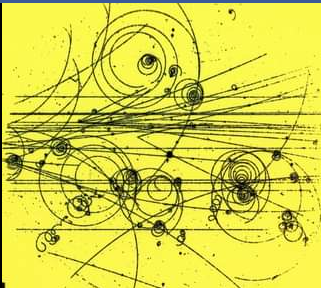# The Yang Mills TheoryThe Yang Mills Theory constitutes one of the most important branches of particle physics, and physics in general. Often considered the cornerstone of theoretical physics, the theory was devised by Chinese physicist Chen Ning Yang and American physicist Robert L. Mills in 1954.

The goal of this theory was to be able to explicitly describe the behaviour of elementary particles linking the electromagnetic force and the weak nuclear force in terms of their geometric structure, or otherwise named quantum field theory (QFT). The main use of QFT is to construct physical models of subatomic particles. The Yang-Mills theory is the basis of our understanding of the Standard Model, and it can be considered a generalization of Maxwell’s unified theory of electromagnetism which encompasses the well known Maxwell Equations. When referring to the Yang Mills Theory, we speak of the respective Yang Mills Equations

With this theory, a contradiction arises between classical and quantum mechanics. The predictions put forward by classical mechanics describe massless particles linked to large range forces. On the other hand, quantum theories must pair massive particles with short range forces. This is how the theory and the understanding of  “mass gaps” was developed.

The strong nuclear force has a mass gap, and this theory specifically concentrates on that mass gap. Once again related to QFT, the mass gap is the difference in energy that exists between the vacuum and the following lowest energy level. The belief is held that the mass gap explains why the strong force is only valid within a small distance range within the atomic nuclie. The experimental evidence for the existence of the mass gaps is present, as it has been demonstrated by various experiments carried out over the decades and likewise in mathematical models; but it remains in the shadows when it comes to any theoretical proof.

The Yang Mills Theory is a non – abelian gauge theory. To understand what this means, we must break down the different definitions included in this phrase. What the gauge theory postulates, in simple words,  is related to the measurement of fundamental fields, which cannot be directly obtained. The field theories can be taken to be indirect representations of what one is actually measuring, by considering the interaction of gauges (examples being charge or velocity). Transformations between the gauges form a group designated as a symmetry group. Mentioning a non – abelian gauge field states that this symmetry group is taken to be non commutative. Nowadays, it can be said that all the gauge fields that are part of the standard model in particle physics can be taken to be Yang Mills fields.

To conclude, one of the interesting parts of the aspects compassed by the Yang Mills theory is that in the current world of physics, it has been designated as a Millenium problem, which encourages scientists to find a solution to be able to establish, through a rigorous mathematical procedure, the existence of the “mass gap” with the reward of a million dollars.

Writer: Maria Esther Vilar Alvarez

Editors: Lamar Albukhari/ Yara Bawazir

REFERENCES

1. Murray, Michael, and Alan Carey. “Millennium Prize: the Yang-Mills Existence and Mass Gap Problem.” The Conversation, 8 Dec. 2011, theconversation.com/millennium-prize-the-yang-mills-existence-and-mass-gap-problem-3848.
2. Hosch, William L. “Yang–Mills Theory.” Encyclopædia Britannica, http://www.britannica.com/science/Yang-Mills-theory.
3. “NLab Yang-Mills Theory.” Yang-Mills Theory in NLab, NLab, ncatlab.org/nlab/show/Yang-Mills+theory.
4. “Yang-Mills Theory.” Yang-Mills Theory – an Overview | ScienceDirect Topics, http://www.sciencedirect.com/topics/physics-and-astronomy/yang-mills-theory.